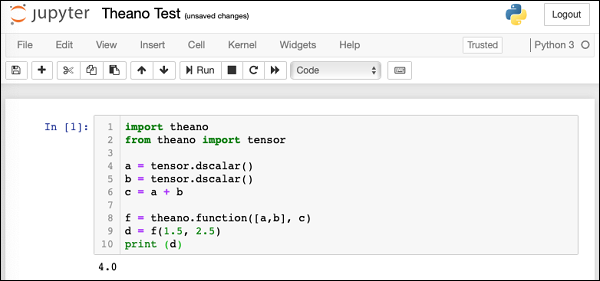# Theano - Installation

Theano can be installed on Windows, MacOS, and Linux. The installation in all the cases is trivial. Before you install Theano, you must install its dependencies. The following is the list of dependencies −

• Python
• NumPy − Required
• SciPy − Required only for Sparse Matrix and special functions
• BLAS − Provides standard building blocks for performing basic vector and matrix operations

The optional packages that you may choose to install depending on your needs are −

• nose: To run Theano’s test-suite
• Sphinx − For building documentation
• Graphiz and pydot − To handle graphics and images
• NVIDIA CUDA drivers − Required for GPU code generation/execution
• libgpuarray − Required for GPU/CPU code generation on CUDA and OpenCL devices

We shall discuss the steps to install Theano in MacOS.

## MacOS Installation

To install Theano and its dependencies, you use pip from the command line as follows. These are the minimal dependencies that we are going to need in this tutorial.

$pip install Theano$ pip install numpy
$pip install scipy$ pip install pydot


You also need to install OSx command line developer tool using the following command −

\$ xcode-select --install


You will see the following screen. Click on the Install button to install the tool.On successful installation, you will see the success message on the console.

## Testing the Installation

After the installation completes successfully, open a new notebook in the Anaconda Jupyter. In the code cell, enter the following Python script −

### Example

import theano
from theano import tensor
a = tensor.dscalar()
b = tensor.dscalar()
c = a + b
f = theano.function([a,b], c)
d = f(1.5, 2.5)
print (d)


### Output

Execute the script and you should see the following output −

4.0


The screenshot of the execution is shown below for your quick reference −If you get the above output, your Theano installation is successful. If not, follow the debug instructions on Theano download page to fix the issues.

## What is Theano?

Now that you have successfully installed Theano, let us first try to understand what is Theano? Theano is a Python library. It lets you define, optimize, and evaluate mathematical expressions, especially the ones which are used in Machine Learning Model development. Theano itself does not contain any pre-defined ML models; it just facilitates its development. It is especially useful while dealing with multi-dimensional arrays. It seamlessly integrates with NumPy, which is a fundamental and widely used package for scientific computations in Python.

Theano facilitates defining mathematical expressions used in ML development. Such expressions generally involve Matrix Arithmetic, Differentiation, Gradient Computation, and so on.

Theano first builds the entire Computational Graph for your model. It then compiles it into highly efficient code by applying several optimization techniques on the graph. The compiled code is injected into Theano runtime by a special operation called function available in Theano. We execute this function repetitively to train a neural network. The training time is substantially reduced as compared to using pure Python coding or even a full C implementation.

We shall now understand the process of Theano development. Let us begin with how to define a mathematical expression in Theano.